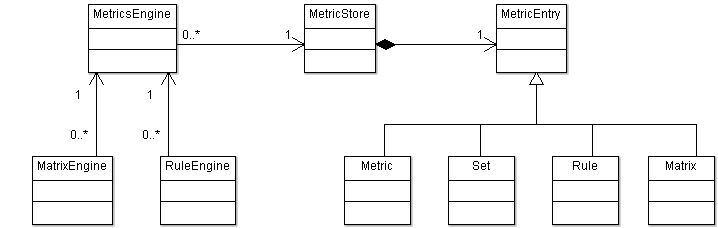www.sdmetrics.com

Package com.sdmetrics.metrics

Provides the core functionality of SDMetrics: calculating metrics, checking design rules.

See: Description

Package com.sdmetrics.metrics Description

Provides the core functionality of SDMetrics: calculating metrics, checking design rules.Tutorial - how to calculate metrics and check design rules for a UML model.

The following code snippets take you through the steps to calculate the design metrics and check the design rules for a UML model, and write the results to the console. We assume we have already loaded the UML model, as described in the tutorial for package com.sdmetrics.model.

1. Required imports:
import java.util.Collection;

import sdmetrics.metrics.Metric;
import sdmetrics.metrics.MetricStore;
import sdmetrics.metrics.MetricsEngine;
import sdmetrics.metrics.Rule;
import sdmetrics.metrics.RuleEngine;
import sdmetrics.metrics.RuleFilter;
import sdmetrics.metrics.RuleViolation;
import sdmetrics.model.MetaModel;
import sdmetrics.model.Model;
import sdmetrics.model.ModelElement;
import sdmetrics.util.XMLParser;

2. Have your model and its metamodel ready (see com.sdmetrics.model), as well as the metric definition file with the metrics and rules you wish to calculate and check, respectively.
MetaModel metaModel = ...;  // metamodel to use
Model model = ...;          // model to uses
String metricsURL = ...;    // metric definition file to use

3. Read the metric definition file
XMLParser parser = new XMLParser();
MetricStore metricStore = new MetricStore(metaModel);
parser.parse(metricsURL, metricStore.getSAXParserHandler());

As is the case for parsing the metamodel and XMI files, you do not have to use the SAX parser provided by class XMLParser. Instances of org.xml.sax.XMLReader that you created yourself will also do.

4. Initialize the metrics engine
MetricsEngine metricsEngine = new MetricsEngine(metricStore, model);

If you only want to check design rules, you can skip the next step and proceed directly with rule checking.

5. Calculate all metrics for all model elements.

In the following nested loop, we only calculate metrics that are not defined as "internal" helper metrics.

for (ModelElement element : model) {
System.out.println("Calculating metrics for element " + element.getFullName());
// calculate and print all metric values for the element
Collection<Metric> metrics = metricStore.getMetrics(element.getType());
for (Metric metric : metrics) {
if (!metric.isInternal()) {
System.out.println("  Metric " + metric.getName() + ": "
+ metricsEngine.getMetricValue(element, metric));
}
}
}

6. Check all rules for all model elements

In this example we use a rule filter to only check design rules for the "analysis" phase. We also skip rules that have been disabled in the metric definition file, and we skip checking rules from which the model element has explicitly been exempted.

RuleEngine ruleEngine = new RuleEngine(metricsEngine);
RuleFilter ruleFilter = new RuleFilter("analysis");

for (ModelElement element : model) {
System.out.println("Checking rules for element " + element.getFullName());
// get rules set of exempted rules for this element
Collection<String> exemptRuleNames = ruleEngine.collectExemptedRules(element);
Collection<Rule> rules = metricStore.getRules(element.getType());
for (Rule rule : rules) {
if (rule.isEnabled() && ruleFilter.match(rule)
&& !exemptRuleNames.contains(rule.getName())) {
List<RuleViolation> issues = ruleEngine.checkRule(element,rule);
for (RuleViolation issue : issues) {
System.out.println("  Violates rule "
+ issue.getRule().getName() + ": "
+ issue.getValue());
}
}
}
}

Instead of manually looping through all model elements, metrics, and rules, you can also use the classes in package com.sdmetrics.app to calculate all data and organize it in a tabular layout.